Maths-
General
Easy

Question

# If the denominator of a certain fraction increased by 1 and the numerator by 2.The fraction becomes equal to 5/8 and if the numerator and denominator are diminished by 1, the fraction becomes equal to 1⁄2. Find the fraction.Hint:

## The correct answer is: 8/15

### Ans :- 8/15 is the value of fraction .Explanation :-Step 1:- Frame the equation based on given conditions.let the  numerator of fraction be x and denominator of fraction is yGiven, If the denominator of a certain fraction increased by 1 and the numerator by 2The fraction becomes 5/8.We get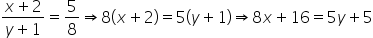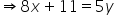— Eq1If the numerator and denominator are diminished by 1, the fraction becomes equal to 1/2.We get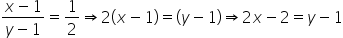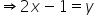— Eq2Step 2:- eliminate the y and find xDoing Eq1-5(Eq2) to eliminate y .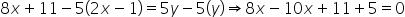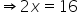∴x = 8 Step 3:- find y by substituting the value of x in eq2.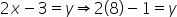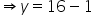∴y = 15 We know the fraction is x/y =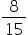#### With Turito Foundation.#### Get an Expert Advice From Turito.